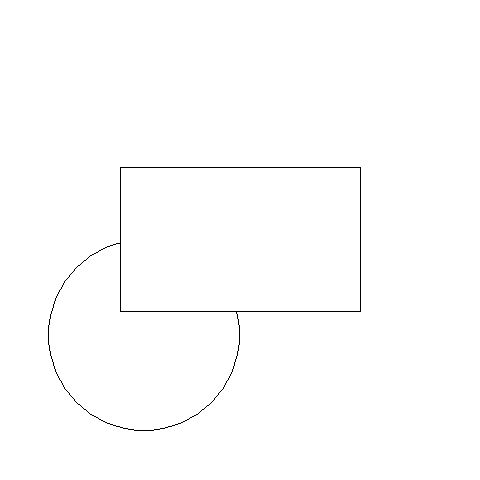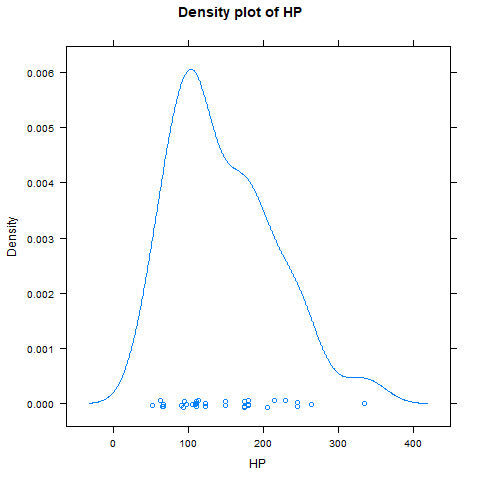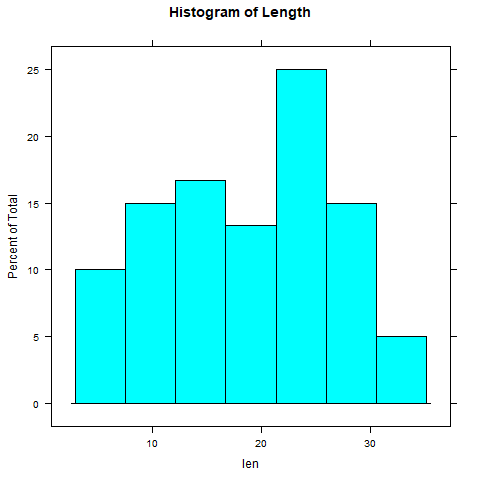# Grid and Lattice Packages in R Programming

Every programming language has packages to implement different functions. Many functions are bundled together in a package. To use those functions, installation and loading of these packages are required. In R programming, there are 10, 000 packages in the CRAN repository. Grid and Lattice are one of the packages in R which implements graphical functions to develop some graphical outputs such as rectangle, circle, histogram, barplot, etc.

#### Grid Package

Grid package in R has been removed from CRAN repository as it is now available as the base package. This package is a basis for all other higher graphical functions used in other packages such as lattice, ggplot2, etc. Also, it can manipulate the lattice outputs. Being a base package, there is no requirement to install it. It is automatically installed when R is installed.
To load the grid package, use below command in console and select “grid” when menu appears-

local({pkg <- select.list(sort(.packages(all.available = TRUE)), graphics=TRUE) + if(nchar(pkg)) library(pkg, character.only=TRUE)})

`grid` package uses functions `circleGrob`, `linesGrob`, `polygonGrob`, `rasterGrob`, `rectGrob`, `segmentsGrob`, `legendGrob`, `xaxisGrob`, and `yaxisGrob` to create graphical objects (grobs).
To see the list of all the functions in `grid` package, use below command-

```library(help = "grid")
```

Below is an implementation of some functions on `grid` package-
Example:

 `library(grid) ` ` `  `# Saving output as png file ` `png(``file` `=``"grid-gfg.png"``) ` ` `  `# Create circle grob ` `cir <``-` `circleGrob(name ``=` `"cir"``, x ``=` `0.3``, y ``=` `0.3``, r ``=` `0.2``, ` `gp ``=` `gpar(col ``=` `"black"``, lty ``=` `3``)) ` ` `  `# Draw grob ` `grid.draw(cir) ` ` `  `# Create rectangular grob ` `rect <``-` `rectGrob(name ``=` `"rect"``, x ``=` `0.5``, y ``=` `0.5``, ` `width ``=` `0.5``, height ``=` `0.3``) ` ` `  `# Draw grob ` `grid.draw(rect) ` ` `  `# Saving the file ` `dev.off() `

Output:#### Lattice Package

The `lattice` package uses `grid` package to provide better relationships between the data. It is an add-on package for implementation of Trellis graphics (graphics that shows relationships between variables conditioned together).
To install this package-

```install.packages("lattice")
```

There are so many graphs present in the package such as barchart, counterplot, densityplot, histogram, etc. A simple format to use is-

```graph_type(formula, data)
```

where,
graph_type
represents the type of graph to represent
formula specifies the variables or conditioned variables

To know about all functions of package-

```library(help = "lattice")
```

Below is an implementation of some of the graphical functions in lattice package-

Example 1:

 `library(lattice) ` ` `  `attach(mtcars) ` ` `  `# Output to be present as PNG file ` `png(``"DensityPlotLatticeGFG.png"``) ` ` `  `# Create Density Plot for HP ` `densityplot(~hp, main ``=``"Density plot of HP"``, xlab ``=``"HP"``) ` ` `  `# Saving the file ` `dev.off() `

Output:Example 2:

 `library(ToothGrowth) ` ` `  `# Output to be present as PNG file ` `png(``"HistogramLatticeGFG.png"``) ` ` `  `# Using ToothGrowth dataset ` `# To Create Histogram of length ` `histogram(~``len``, data ``=` `ToothGrowth, ` `main ``=` `"Histogram of Length"``) ` ` `  `# Saving the file ` `dev.off() `

Output:My Personal Notes arrow_drop_upBlockchain Enthusiast

If you like GeeksforGeeks and would like to contribute, you can also write an article using contribute.geeksforgeeks.org or mail your article to contribute@geeksforgeeks.org. See your article appearing on the GeeksforGeeks main page and help other Geeks.

Please Improve this article if you find anything incorrect by clicking on the "Improve Article" button below.

Article Tags :

Be the First to upvote.

Please write to us at contribute@geeksforgeeks.org to report any issue with the above content.